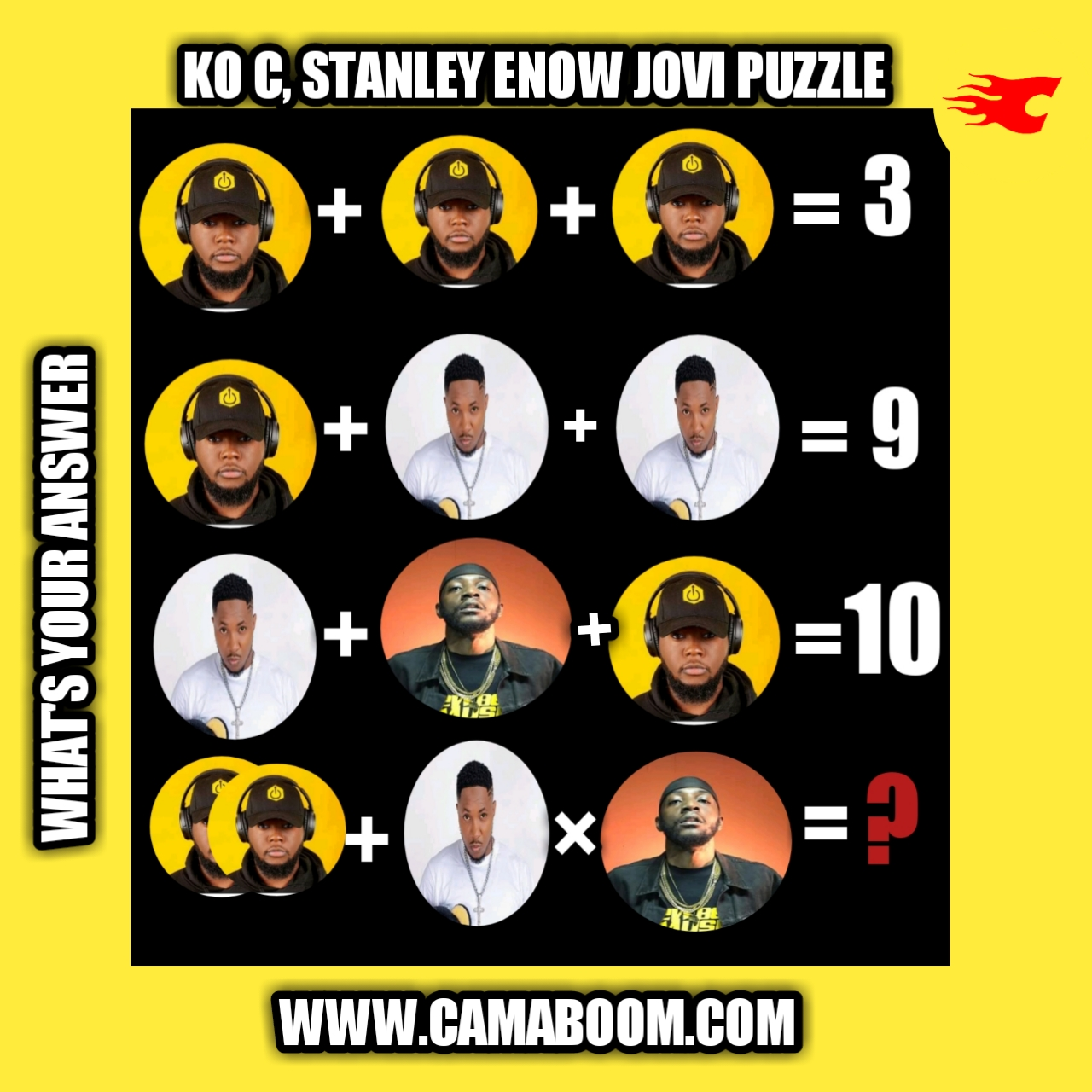0# Ko-C , Stanley Enow Jovi Puzzle answer

This Cameroonian Music singers math  puzzle is making waves over the internet. It looks simple at a first glance but many who attempted to solve it get the wrong answer first. Majority of people who tried  to solve it had 13 while some had 22. Today I will solve the KO-C, Stanley Enow and Jovi Puzzle for you in detail.

Ko-C, Stanley Enow and Jovi are top rappers in Cameroon. It's important to note that this is not a  quiz to compare artists but just a puzzle to get you busy, relax your brain and show your mathematical skills.

2KO-C +  Stanley Enow Times Jovi = ?

The puzzle is tricky at the end so you have to follow my solving step by step.

Solution:

From the picture above, I will form four equations, solve equation one to three then substitute their values in the final equation.

Equation One: 3 Ko-C = 3,

Implies Ko-C = 3÷3

Implies Ko-C = 1.

Equation Two: Ko-C + Stanley Enow + Stanley =9.

Implies 2Stanley Enow = 8,

Stanley Enow = 8÷2,

Stanley Enow = 4.

Equation Three: Stanley Enow +Jovi + Ko-C = 10,

Implies 4+Jovi + 1 = 10.

Implies  Jovi = 10 - 5

Jovi = 5.

Equation four: Final equation.

From the solving above,

Ko-C = 1,

Stanley Enow = 4

Jovi = 5.

I will substitute the values of each rapper in the final equation and simplify as thus:

Here's the final equation again

2KO-C + Stanley Enow × Jovi = ?.

2(1) + 4 ×5 =?

2+4×5=?

2+20=? ( Apply BODMAS ),

22=?.

Therefore the final Answer is 22. If you have 13 as your Answer, it means you didn't apply BODMAS well. If you have any questions, comment below.

Tags